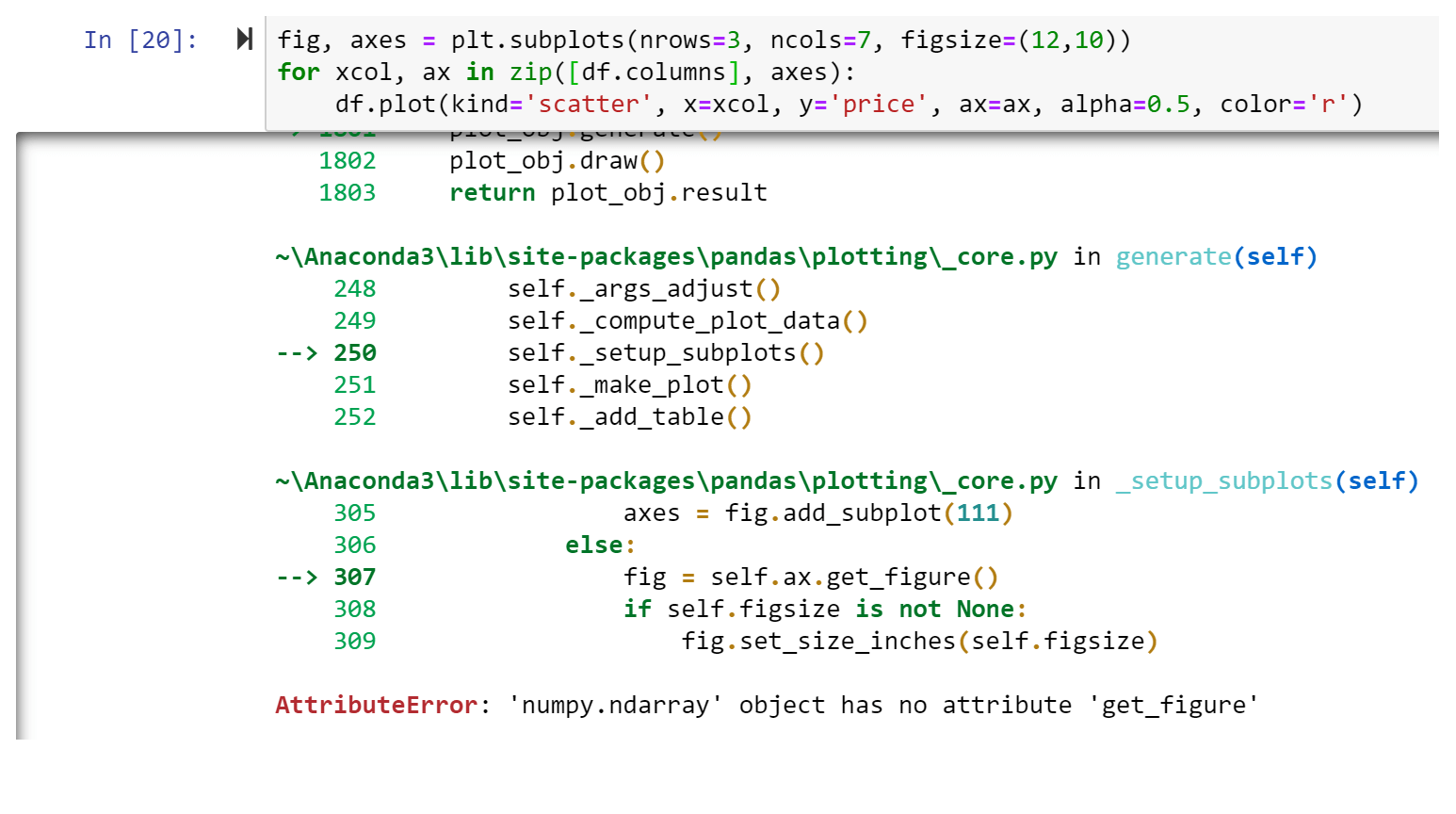# Cloud Stack Ninja

I'm an encountering an issue when I try to run a cell of code. I'm attempting to make a scatter plot for each of the variables in my dataframe, but am encountering an error i'm not too sure about. Can you help?

My code:

``````fig, axes = plt.subplots(nrows=3, ncols=7, figsize=(12,10))
for xcol, ax in zip(df[df.columns], axes):
df.plot(kind='scatter', x=xcol, y='price', ax=ax, alpha=0.5, color='r')
``````

Returned error: AttributeError: 'numpy.ndarray' object has no attribute 'get_figure'